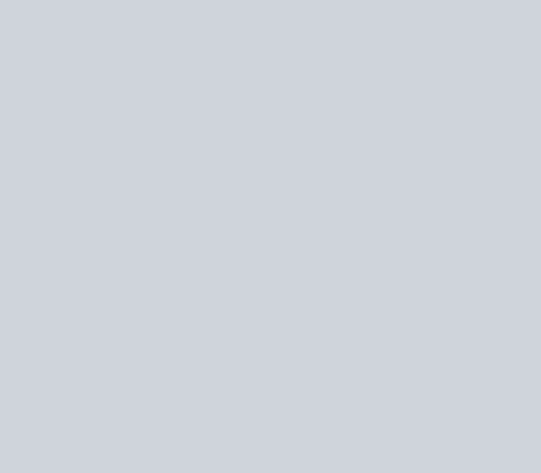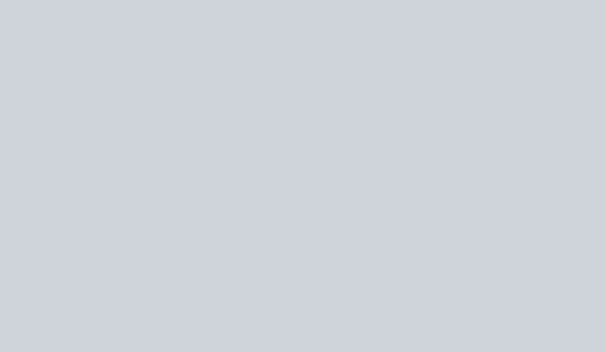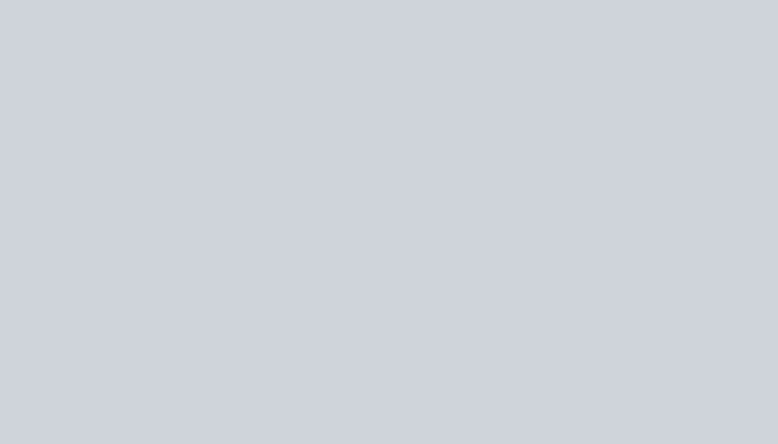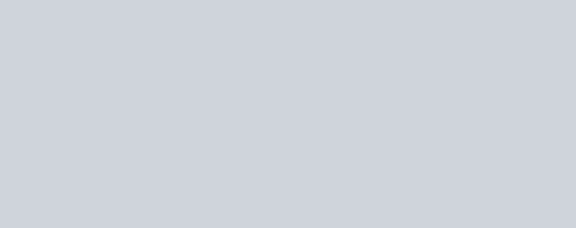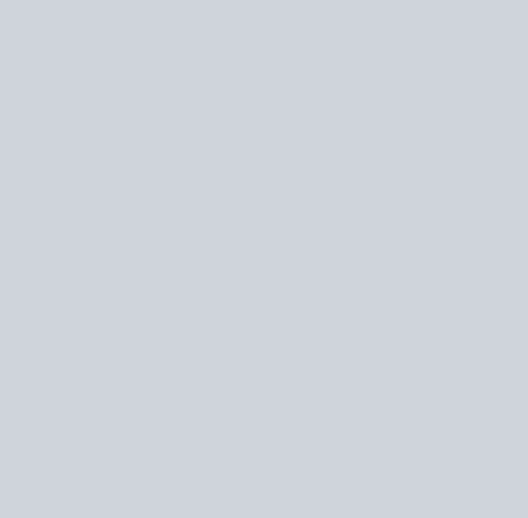Sketch the region common to the circle
Question:

Sketch the region common to the circle $x^{2}+y^{2}=16$ and the parabola $x^{2}=6 y$. Also, find the area of the region, using integration.

Solution: